# Simplifying Ratios Worksheet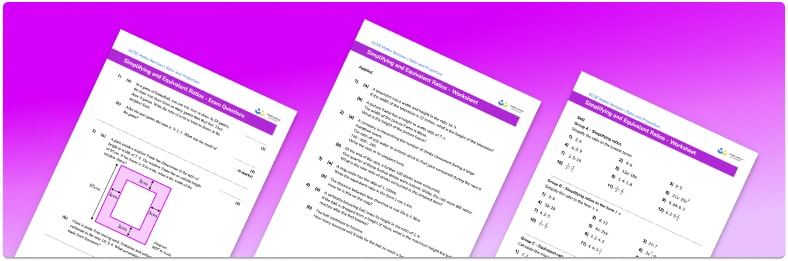• This field is for validation purposes and should be left unchanged.

You can unsubscribe at any time (each email we send will contain an easy way to unsubscribe). To find out more about how we use your data, see our privacy policy.

• Section 1 of the simplifying ratios worksheet contains 36 skills-based simplifying ratios questions, in 3 groups to support differentiation
• Section 2 contains 4 applied simplifying ratios questions with a mix of worded problems and deeper problem solving questions
• Section 3 contains 4 foundation and higher level GCSE exam style simplifying ratios questions
• Answers and a mark scheme for all simplifying ratios questions are provided
• Questions follow variation theory with plenty of opportunities for students to work independently at their own level
• All questions created by fully qualified expert secondary maths teachers
• Suitable for GCSE maths revision for AQA, OCR and Edexcel exam boards

### Simplifying ratios at a glance

A ratio compares how much there is of one thing in relation to another. When writing ratios, we can often simplify them by dividing each part by a common factor. Writing ratios in their simplest form makes it easier to visualise the relationship between the quantities in the ratios. It also makes it easier to use the ratios. You should be able to simplify two part and three part ratios. When simplifying a ratio, the ratio should contain integers (whole numbers).

Sometimes we might want to write ratios in the form 1:n where n is the unit rate. A good example of this is when writing the scale of a map. This is done by dividing each part of the ratio by a common amount.

When writing and simplifying ratios, we need to ensure that we use the same units for each part of the ratio.

We can calculate equivalent ratios by multiplying each part by the same number. Equivalent ratios are useful to solve problems.

Looking forward, students can then progress to additional ratio and proportion worksheets, for example aor a direct proportion worksheet.

For more teaching and learning support on Ratio and Proportion our GCSE maths lessons provide step by step support for all GCSE maths concepts.

## Related worksheets

Ratio To Fraction Worksheet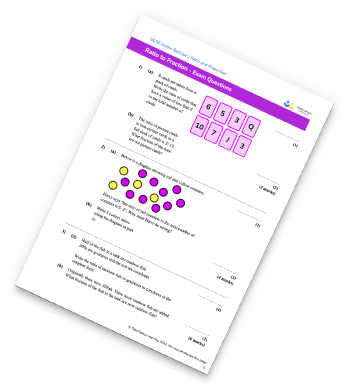Ratio Worksheet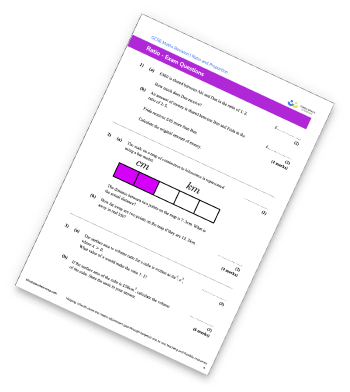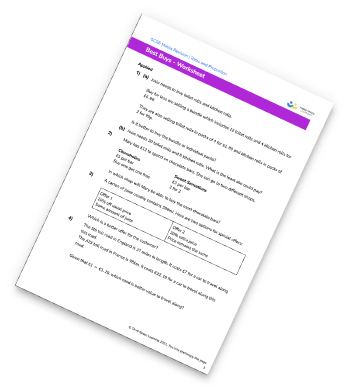Proportion Worksheet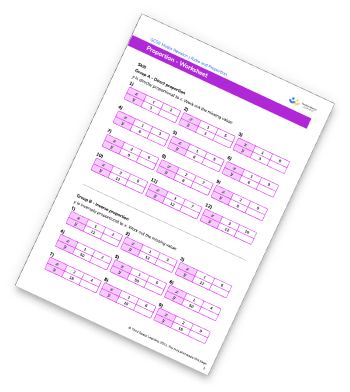## Do you have KS4 students who need more focused attention to succeed at GCSE?There will be students in your class who require individual attention to help them succeed in their maths GCSEs. In a class of 30, it’s not always easy to provide.

Help your students feel confident with exam-style questions and the strategies they’ll need to answer them correctly with our dedicated GCSE maths revision programme.

Lessons are selected to provide support where each student needs it most, and specially-trained GCSE maths tutors adapt the pitch and pace of each lesson. This ensures a personalised revision programme that raises grades and boosts confidence.

Find out more